#### You may also like### Have You Got It?

Can you explain the strategy for winning this game with any target?### Counting Factors

Is there an efficient way to work out how many factors a large number has?### Is There a Theorem?

Draw a square. A second square of the same size slides around the first always maintaining contact and keeping the same orientation. How far does the dot travel?

# Shear Magic

##### Age 11 to 14Challenge Level

Shape C is a parallelogram with a base of 2 and a height of 3.
Can you use the two pictures below to work out the area of the parallelogram?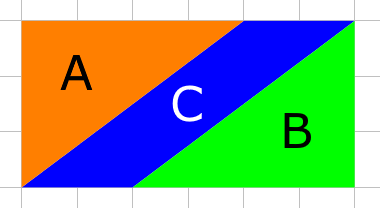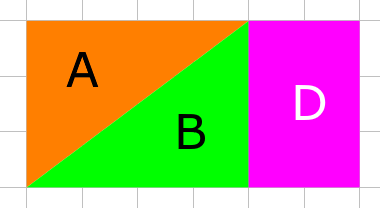Here are two more parallelograms made by shearing a rectangle with a base of 2 and a height of 3.
Can you draw similar diagrams to work out their areas?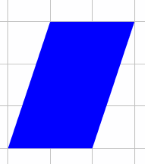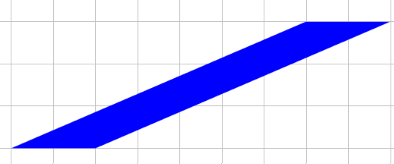Use the GeoGebra applet below to explore other parallelograms with a base of 2 and a height of 3.
What do you notice? Can you explain it?

Explore other families of parallelograms with a particular base and height, by moving the red and green dots.

Can you come up with a general rule for working out the area of a parallelogram if you know its base and height? Can you explain why your rule works?

I wonder what happens when we shear triangles...
Here is a family of four sheared triangles with a base of 1 and a height of 2.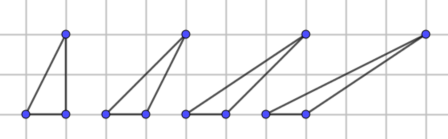Can you work out the area of the triangles?
You might like to use the GeoGebra applet below to help.

Explore other families of triangles with a particular base and height.

Can you come up with a general rule for working out the area of a triangle if you know its base and height?

Can you explain why your rule works?

Notes and Background

Shearing is a transformation of a shape in which a particular line (in this case the base of the triangle or parallelogram) remains fixed and all other points in the shape are translated parallel to that line by an amount proportional to the distance from that line.